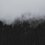# Introduction to the new decade 2020 !

To start a new year with the love for math and science, I made a funny solving of a "Today's" problem. Disliking it counts as a like to me :P . For the introduction of the new year:

## You can skip this note if you are not into jokes.

The following steps explain the solving process of the problem (1 Jan 2020) [it is about the no. 2020 we ae doing math with]:

We want to express 2020 in the form of $a^{2} -b^{2}$ :

• $2020 = a^{2} -b^{2} = (a+b) \times (a-b)$
• Since these are powers, we can use the $Yeet.theorem$ to yeet over the exponent: $2020 = 2 \times a -2 \times b$
• $1010 = (a - b)$
• We repalce $(a-b)$ with 1010 at eq.1 which we get:
• $2020 = (a+b) \times (1010)$
• $2 = (a+b)$ divide both sides by 1010

We have the given system to solve: $\begin{cases} 2 = (a+b) \\ 1010 = (a - b) \end{cases}$/extract_itex] • $1012 = (a + b) + (a - b)$ • $1012 = 2 \times a$ • $506 = a$ Finding $b$: • $1010 = (506 - b)$ • $b = - 504$ But we cannot have negative numbers so $b = 504$. We have solution that $\boxed{2020 = 506^{2} -504^{2}}$Note by Nikolas Кraj 11 months ago This discussion board is a place to discuss our Daily Challenges and the math and science related to those challenges. Explanations are more than just a solution — they should explain the steps and thinking strategies that you used to obtain the solution. Comments should further the discussion of math and science. When posting on Brilliant: • Use the emojis to react to an explanation, whether you're congratulating a job well done , or just really confused . • Ask specific questions about the challenge or the steps in somebody's explanation. Well-posed questions can add a lot to the discussion, but posting "I don't understand!" doesn't help anyone. • Try to contribute something new to the discussion, whether it is an extension, generalization or other idea related to the challenge. • Stay on topic — we're all here to learn more about math and science, not to hear about your favorite get-rich-quick scheme or current world events. MarkdownAppears as *italics* or _italics_ italics **bold** or __bold__ bold - bulleted- list • bulleted • list 1. numbered2. list 1. numbered 2. list Note: you must add a full line of space before and after lists for them to show up correctly paragraph 1paragraph 2 paragraph 1 paragraph 2 [example link](https://brilliant.org)example link > This is a quote This is a quote  # I indented these lines # 4 spaces, and now they show # up as a code block. print "hello world" # I indented these lines # 4 spaces, and now they show # up as a code block. print "hello world" MathAppears as Remember to wrap math in $$ ... $$ or \[ ... $ to ensure proper formatting.
2 \times 3 $2 \times 3$
2^{34} $2^{34}$
a_{i-1} $a_{i-1}$
\frac{2}{3} $\frac{2}{3}$
\sqrt{2} $\sqrt{2}$
\sum_{i=1}^3 $\sum_{i=1}^3$
\sin \theta $\sin \theta$
\boxed{123} $\boxed{123}$

Sort by:

Let's list all things happened in 2020: Covid19; coffin dance meme; Fall guys become popular; Among us gets popular; Trump gets Covid19; Random Covid; More Covid lol

- 1 month, 2 weeks ago

You forgot that the sun is getting larger due to the giant phase too and the Nazi zombie outbreak

- 1 month, 2 weeks ago

YEET! lol

- 1 month, 2 weeks ago

Looks like this year yeeted us as well. Yeet.

- 6 months, 2 weeks ago

I don't get the Yeet Theorem well yeet.

- 11 months ago

It is a joke; a meme, that represents a 'proof' of a theorem:

- 11 months ago

It was just a pun. But thank you anyway.

- 11 months ago

This is misuse of the Yeet Theorem. The Yeet theorem states that you can yeet the exponents to the end of the equation.

- 11 months ago

Just saying, this is a joke, I'm not trying to be mean in any way.

- 6 months, 2 weeks ago

It's even better that it actually works! Good joke.

- 11 months ago

Good one!

"Yeet Theorem" I'm dying

- 11 months ago

This is Funny!

- 11 months ago